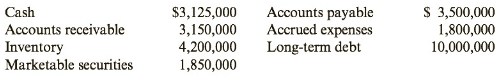Chapter 8, Problem 34CE### Cornerstones of Financial Accounti...

4th Edition
Jay Rich + 1 other
ISBN: 9781337690881

#### Solutions

Chapter
Section### Cornerstones of Financial Accounti...

4th Edition
Jay Rich + 1 other
ISBN: 9781337690881
Textbook Problem
1 views

# Liquidity RatiosGER’s financial statements contain the following information:Note: Round answers to two decimal places.Required:1. What is the current ratio?2. What is the quick ratio?3. What is the cash ratio?4. Discuss GER’s liquidity using these ratios.

To determine

Concept introduction:

Current Ratio:

Current Ratio is measure of the company’s ability to pay off its current liabilities using its current assets. It is calculated by dividing the total current assets by total current liabilities. The formula of the current ratio is as follows:

Current Ratio=Current assetsCurrent liabilities

Acid test ratio:

Acid test ration is also called Quick ratio. This ratio is calculated by dividing the quick assets (Cash, Cash equivalents, Short term investments and current receivables) by total current liabilities for the year. The formula for Acid test ratio is as follows:

Acid test ratio = (Cash + Cash equivalents + Short term investments + Accounts receivables) Current Liabilities

Cash ratio:

Cash ratio is calculated by dividing and cash and cash equivalents by the total current liabilities. The formula for Cash ratio is as follows:

Cash Ratio = Cash and cash equivalents/ Current liabilities

Requirement 1:

To calculate:

The Current Ratio.

Explanation

The Current Ratio is calculated as follows:

 Cash $3,125,000 Marketable Securities$ 1,850,000 Accounts Receivables $3,150,000 Inventory$ 4,200,000 Total Current Assets (A) \$ 12,325,0...
To determine

Concept introduction:

Current Ratio:

Current Ratio is measure of the company’s ability to pay off its current liabilities using its current assets. It is calculated by dividing the total current assets by total current liabilities. The formula of the current ratio is as follows:

Current Ratio=Current assetsCurrent liabilities

Acid test ratio:

Acid test ration is also called Quick ratio. This ratio is calculated by dividing the quick assets (Cash, Cash equivalents, Short term investments and current receivables) by total current liabilities for the year. The formula for Acid test ratio is as follows:

Acid test ratio = (Cash + Cash equivalents + Short term investments + Accounts receivables) Current Liabilities

Cash ratio:

Cash ratio is calculated by dividing and cash and cash equivalents by the total current liabilities. The formula for Cash ratio is as follows:

Cash Ratio = Cash and cash equivalents/ Current liabilities

Requirement 2:

To calculate:

The Quick Ratio.

To determine

Concept introduction:

Current Ratio:

Current Ratio is measure of the company’s ability to pay off its current liabilities using its current assets. It is calculated by dividing the total current assets by total current liabilities. The formula of the current ratio is as follows:

Current Ratio=Current assetsCurrent liabilities

Acid test ratio:

Acid test ration is also called Quick ratio. This ratio is calculated by dividing the quick assets (Cash, Cash equivalents, Short term investments and current receivables) by total current liabilities for the year. The formula for Acid test ratio is as follows:

Acid test ratio = (Cash + Cash equivalents + Short term investments + Accounts receivables) Current Liabilities

Cash ratio:

Cash ratio is calculated by dividing and cash and cash equivalents by the total current liabilities. The formula for Cash ratio is as follows:

Cash Ratio = Cash and cash equivalents/ Current liabilities

Requirement 3:

To calculate:

The Cash Ratio.

To determine

Concept introduction:

Current Ratio:

Current Ratio is measure of the company’s ability to pay off its current liabilities using its current assets. It is calculated by dividing the total current assets by total current liabilities. The formula of the current ratio is as follows:

Current Ratio=Current assetsCurrent liabilities

Acid test ratio:

Acid test ration is also called Quick ratio. This ratio is calculated by dividing the quick assets (Cash, Cash equivalents, Short term investments and current receivables) by total current liabilities for the year. The formula for Acid test ratio is as follows:

Acid test ratio = (Cash + Cash equivalents + Short term investments + Accounts receivables) Current Liabilities

Cash ratio:

Cash ratio is calculated by dividing and cash and cash equivalents by the total current liabilities. The formula for Cash ratio is as follows:

Cash Ratio = Cash and cash equivalents/ Current liabilities

Requirement 4:

To discuss:

Liquidity of the company.

### Still sussing out bartleby?

Check out a sample textbook solution.

See a sample solution

#### The Solution to Your Study Problems

Bartleby provides explanations to thousands of textbook problems written by our experts, many with advanced degrees!

Get Started

#### What does the term 3 Vs refer to?

Database Systems: Design, Implementation, & Management

#### Explain what is meant by modulus of elasticity and modulus of rigidity.

Engineering Fundamentals: An Introduction to Engineering (MindTap Course List)

#### Define polymorphism, and provide three examples.

Systems Analysis and Design (Shelly Cashman Series) (MindTap Course List)

#### A good practice to secure your wireless network is to immediately broadcast the SSID.

Enhanced Discovering Computers 2017 (Shelly Cashman Series) (MindTap Course List)